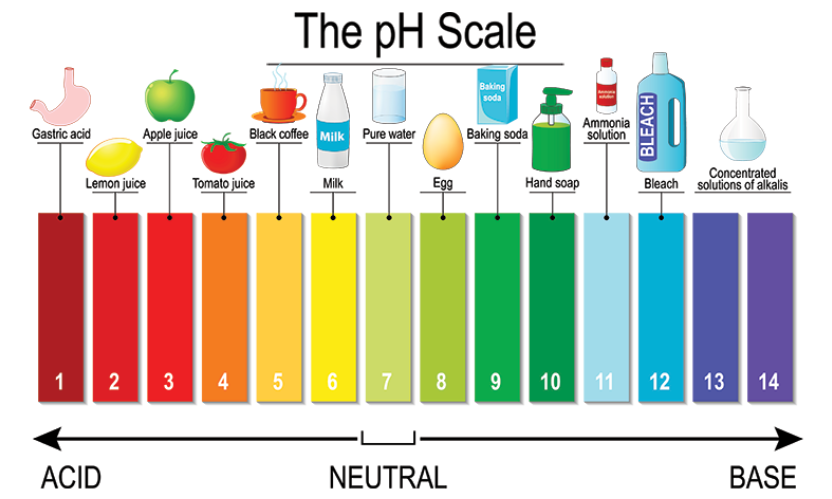# Why does distilled water not conduct electricity, whereas rainwater does?

Distilled water does not conduct electricity because it is pure water that is free from all the ionic species.

Rainwater, while falling to the earth through the atmosphere, dissolves an acidic gas carbon dioxide from the air and forms carbonic acid (H2CO3). Carbonic acid provides hydrogen ions, H+(aq), and carbonate ions, CO32- (aq), to rainwater. So, due to the presence of carbonic acid (which provides ions to rain water), the rain water conducts electricity.

[Extra information:The pH of a solution is a measure of the molar concentration of hydrogen ions in the solution and as such is a measure of the acidity or basicity of the solution.

The letters pH stand for "power of hydrogen" and the numerical value for pH is just the negative of the power of 10 of the molar concentration of H+ ions.

To calculate the pH of an aqueous solution you need to know the concentration of the hydronium ion in moles per litre (molarity).

The pH is then calculated using the expression: pH = - log [H3O+].Updated on: 14-Mar-2023

41 Views